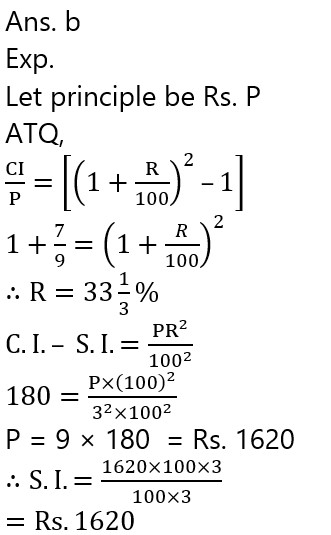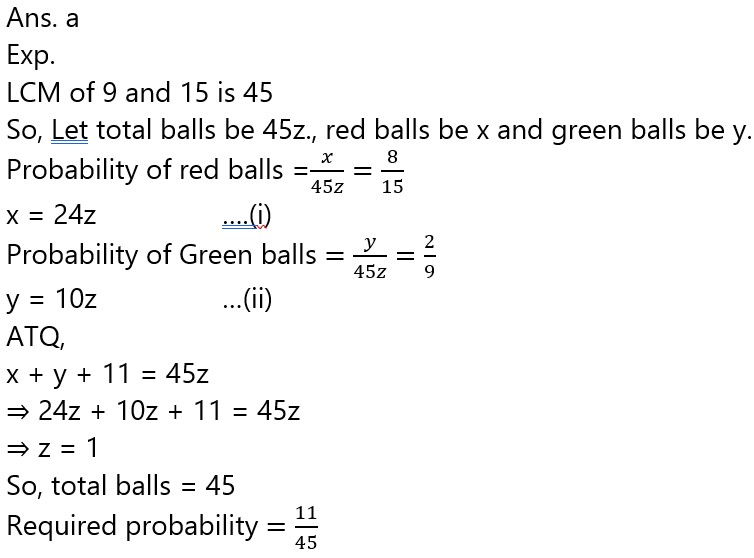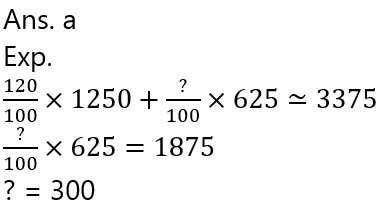# SBI PO Prelims 2022 Quantitative Aptitude Quiz- 23

## SBI PO Prelims Quantitative Aptitude Quiz

Quantitative Aptitude is the most significant part of almost all competitive exams. Candidates appear to be having difficulty with Quantitative Aptitude Questions, so they need to practice more. Even if the portion is difficult, if you practice enough questions and learn the basic concepts, you can surely do well. In this article, we have come up with the SBI PO Prelims Quantitative Aptitude Quiz to enhance your preparation. This SBI PO Prelims Quantitative Aptitude Quiz will assist you to cope with the tough competition. This SBI PO Prelims Quantitative Aptitude Quiz is available to you at no cost. Through this SBI PO Prelims Quantitative Aptitude Quiz, you will learn formulas and short tricks to solve the Quantitative Aptitude Questions. Candidates must solve this SBI PO Prelims Quantitative Aptitude Quiz to improve their exam preparation.

1. Two friend Shantanu and Aarav enter into a business investing in ratio 3 : 5. Ratio of time period for the investment of Aarav and Shantanu is 2 : 3 and profit earned by Aarav is Rs. 450 more than Shantanu. If another friend Ashish had profit which is 40% of the total profit earned by both then find profit of Ashish ?

(a) Rs. 3420

(b) Rs. 3240

(c) Rs. 3560

(d) Rs. 2800

(e) None of these1. If the ratio of compound interest and principle for two years is 7 : 9 and the difference between CI and SI for same period is Rs 180. Then find the simple interest on same principle for three years at same rate ?

(a) Rs. 1440

(b) Rs. 1620

(c) Rs. 1820

(d) Rs. 1280

(e) Rs. 16401. The ratio of height and radius of a cylinder is 2 : 5 and diameter of cylinder is equal to the diagonal of square whose area is 400 m². Then find the volume of cylinder (in m³)1. A sack contains some red, blue and green color balls. The probability of selecting one red ball out of total balls is 8/15 and the probability of selecting one green ball out of total balls is 2/9. The number of blue colour balls in the sack is 11. Find probability of selecting one blue ball?

(a)  11/45

(b)  12/35

(c)  14/45

(d)  13/35

(e)  17/351. An article is sold at 40% discount on M.P. and ratio of C.P. to S.P. is 5 : 8 then what is the M.P. of article if the profit obtained on same article is Rs. 450.

(a) Rs. 1400

(b) Rs. 2400

(c) Rs. 1600

(d) Rs. 2200

(e) Rs. 2000Directions (6-10): What approximate value will come in place of  (?) in the following questions ?

6.119.83% of 1249.93 + ? % of 624.89 = 3374.92

(a) 300

(b) 350

(c) 400

(d) 425

(e) 2507.      20.02 × 32.92 + √(63.86)  × 9.89 = 20.01 × √(?)

(a) 1469

(b) 1423

(c) 1253

(d) 1369

(e) 1163

Ans. d

Exp.

20 × 33 + √64  × 10 ≃ 20 × √(?)

660 + 80 ≃ 20 × √(?)

√(?)≃740/20=37

? = 1369

1. 2898.98 ÷ 289.92 + 19.98 + (?)² = 93.98

(a) 12

(b) 6

(c) 8

(d) 14

(e) 101. 29.8% of 701 – 350.01 + 82% of 501 = ?

(a) 230

(b) 330

(c) 370

(d) 270

(e) 2101. 1519.98 + 179.7 × 20.01 + ? = (1.99) × 9.8

(a) 10

(b) 5

(c) 15

(d) 20

(e) 0